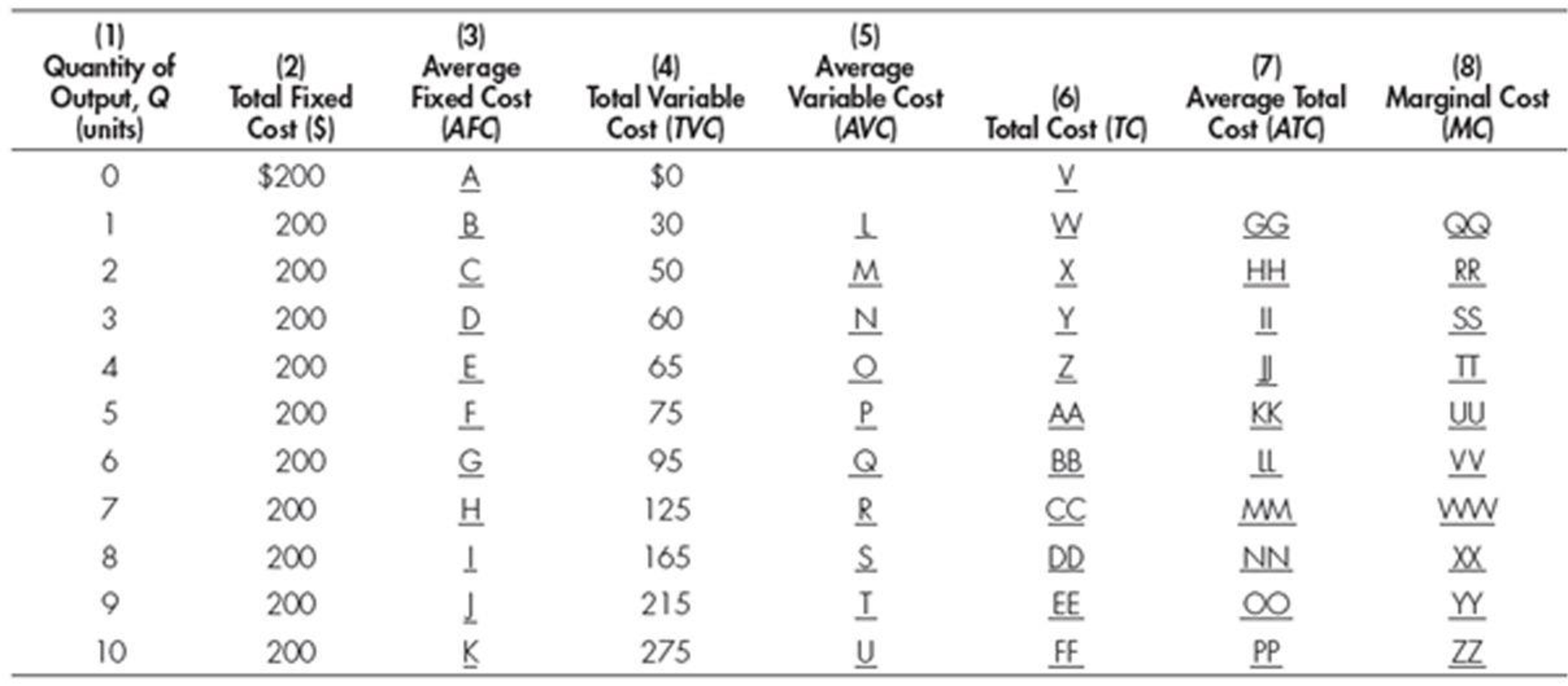Chapter 21, Problem 1WNG

Chapter
Section
Textbook Problem

For each lettered space in the following table, determine the appropriate dollar amount:To determine

Illustrate the value of average fixed cost, average variable cost, total cost, average total cost, and marginal cost.

Explanation

Average fixed cost:

Average fixed cost can be calculated using the following formula:

Average fixed  cost=(Total fixed costQuantity)        (1)

Average fixed cost:

If fixed cost is 200 and the quantity is 1, then the average fixed cost can be calculated using Equation (1) as follows:

Average fixed  cost=(Total fixed costQuantity)=2001=200

Thus, if output is 1, then the value of average fixed cost is $200. Average variable cost: Average variable cost can be calculated using the following formula: Average variable cost=(Total variable costQuantity) (2) Average variable cost: If total variable cost is 30 and the quantity is 1, then the average variable cost can be calculated using Equation (2) as follows: Average variable cost=(Total variable costQuantity)=301=30 Thus, if the output is 1, then the value of average variable cost is$30.

Total cost:

Total cost can be calculated using the following formula:

Total cost=Toal fixed cost+Total variable cost        (3)

Total cost:

If total variable cost is 30 and total fixed cost is 200, then the total cost can be calculated using Equation (3) as follows:

Total cost=Toal fixed cost+Total variable cost=200+30=230

Thus, if output is 1, then the value of total cost is \$230

Still sussing out bartleby?

Check out a sample textbook solution.

See a sample solution

The Solution to Your Study Problems

Bartleby provides explanations to thousands of textbook problems written by our experts, many with advanced degrees!

Get Started

What is an assets depreciable cost?

College Accounting, Chapters 1-27 (New in Accounting from Heintz and Parry)

Explain the following statement: Our tax rates are progressive.

Fundamentals of Financial Management (MindTap Course List)

STOCK REPURCHASES Beta Industries has net income of 2,000,000, and it has 1,000,000 shares of common stock outs...

Fundamentals of Financial Management, Concise Edition (with Thomson ONE - Business School Edition, 1 term (6 months) Printed Access Card) (MindTap Course List)